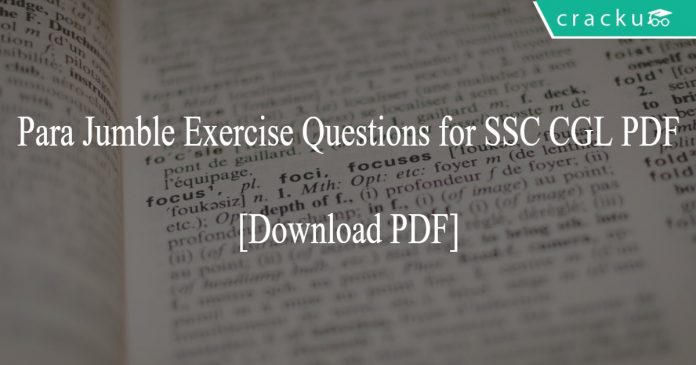# Para Jumble Exercise Questions for SSC CGL PDF

0
3247Para Jumble Exercise Questions for SSC CGL PDF

# Para Jumble Exercise Questions for SSC CGL PDF:

Download Important practice set for SSC CGL exam exercise test questions based on previous SSC asked papers. Sentence Arrangement questions and answers with detailed solutions.

[icegram campaigns=”11442″]

Instructions:

Rearrange the following sentences (A), (B), (C), (D), (E) and (F) to make a meaningful paragraph and then answer the questions which follow

(A) While these disadvantages of bio fuels are serious, they are the only alternate energy source of the future and the sooner we find solutions to these problems the faster we will be able to solve the problems wo are now facing with gasoline.

(B) This fuel can also help to stimulate jobs locally since they are also much safer to handle thaw gasoline and can thus have the potential to turnaround a global economy.

(C) These include dependence on fossil fuels for the machinery required to produce biofuel which ends up polluting as much as the burning of fossil fuels on roads and exorbitant cost of biofuels which makes it very difficult for the common man to switch to this option.

(D) This turnaround can potentially help to bring world peace and end the need to depend on foreign countries for energy requirements.

(E) Biofuels are made from plant sources and since these sources are available in abundance and can be reproduced on a massive scale they form an energy source that is potentially unlimited.

(F) However everything is not as green with the biofuels as it seems as there are numerous disadvantages involved which at times overshadow their positive impact.

Question 1:

Which of the following sentence should be the FIFTH after rearrangement ?

a) A
b) B
c) C
d) E
e) F

Question 2:

Which of the following sentence should be the THIRD after rearrangement ?

a) A
b) B
c) C
d) D
e) E

Question 3:

Which of the following sentence should be the FIRST after rearrangement ?

a) A
b) B
c) C
d) D
e) E

Question 4:

Which of the following sentence should be the SIXTH (LAST) after rearrangement ?

a) A
b) C
c) D
d) E
e) F

Question 5:

Which of the following sentence should be the SECOND after rearrangement ?

a) A
b) B
c) D
d) E
e) F

Solutions (1 to 5)

1) Answer (c)

The correct order of sentences is as follows: EBDFCA. E opens the paragraph with an introduction to biofuels. B talks about the advantages of these fuels. The use of the words “this turnaround” in sentence D is a reference to the turnaround talked about in sentence B. So, D follows B. The next sentence is F, which is followed by C and then A is the concluding statement.
So, statement C is the fifth sentence.

2) Answer (d)

The correct order of sentences is as follows: EBDFCA. E opens the paragraph with an introduction to biofuels. B talks about the advantages of these fuels. The use of the words “this turnaround” in sentence D is a reference to the turnaround talked about in sentence B. So, D follows B. The next sentence is F, which is followed by C and then A is the concluding statement.
D is the third sentence after rearrangement.

3) Answer (e)

The correct order of sentences is as follows: EBDFCA. E opens the paragraph with an introduction to biofuels. B talks about the advantages of these fuels. The use of the words “this turnaround” in sentence D is a reference to the turnaround talked about in sentence B. So, D follows B. The next sentence is F, which is followed by C and then A is the concluding statement.
E is the first statement after rearrangement.

4) Answer (a)

The correct order of sentences is as follows: EBDFCA. E opens the paragraph with an introduction to biofuels. B talks about the advantages of these fuels. The use of the words “this turnaround” in sentence D is a reference to the turnaround talked about in sentence B. So, D follows B. The next sentence is F, which is followed by C and then A is the concluding statement.
A is the last sentence after rearrangement.

5) Answer (b)

The correct order of sentences is as follows: EBDFCA. E opens the paragraph with an introduction to biofuels. B talks about the advantages of these fuels. The use of the words “this turnaround” in sentence D is a reference to the turnaround talked about in sentence B. So, D follows B. The next sentence is F, which is followed by C and then A is the concluding statement.
B is the second sentence after rearrangement.